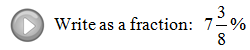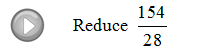## Pages

Showing posts with label fractions. Show all posts
Showing posts with label fractions. Show all posts

### Review of Decimals and Percents

Percents are an important part of our everyday life and will show up often in our study of Algebra.  You will have to know how to convert percents to real numbers and back again.
Using the definition you can convert percentages to fractions very easily. Write the number over 100 and eliminate the percent symbol %, then reduce if necessary.
To find a decimal equivalent divide.
Notice that you could get the same result by moving the decimal over 2 places to the left.
This works because a percent is part of 100 and dividing by 100 simply moves the decimal two places.
Rewrite as a decimal.
To write a decimal as a percent, you can multiply by 1 = 100%. For example,
This can be done quickly by moving the decimal to the right two places.  One way to remember how to convert between decimals and percents is to use the letters D and P in alphabetical order and the following illustration.
If you have a decimal the illustration shows to move the decimal two places to the right. If you have a percent the illustration shows to move the decimal two places to the left.

Re-write as a percent.
When converting percents to fractions there really is no easy trick.  The idea is to use the definition and then reduce.  You must remember that a percent is a part of 100.
Re-write as fractions.
Typical Word Problems:
Tax - A calculator is advertised at \$12.99 plus a 7.5% sales tax.  What is the total including tax?
Tip - After a nice dinner out, the bill came to \$35.75.  For good service you wish to leave a 15% tip.  What is the total including the tip?

Video Examples on YouTube:### Fractions

Fractions can be a barrier to beginning algebra students.  Also referred to as rational numbers, fractions are simply real numbers that can be written as a quotient, or ratio, of two integers.
Equivalent fractions can be expressed with different numerators and denominators. For example,
If you eat 4 out of 8 slices of pizza, that is the same as eating one-half of the pie.  Usually we will be required to reduce fractions to lowest terms. Fractions in lowest terms have no common factors in the numerator and denominator other than 1.
An alternative and more common method of reducing is to identify the greatest common factor (GCF) of the numerator and denominator and then divide both by that number.
An improper fraction is one where the numerator is larger than the denominator.  To convert an improper fraction to a mixed number, simply divide. The quotient is the whole number part and the remainder is the new numerator.
Reduce.
To multiply fractions, you multiply the numerators and the denominators. NO COMMON DENOMINATOR is required.
To divide fractions, you multiply the numerator by the reciprocal of the divisor.
When multiplying, look for factors to cancel before actually multiplying the numerators and denominators.  This will eliminate the need to reduce the end result.

Multiply or Divide.
Convert mixed numbers to improper fractions before you multiply or divide. To do this, multiply the denominator with the whole number then add the numerator, this will be the new numerator.
Divide.

Sometimes mixed numbers are confused with multiplication.  Be sure to remember that 4 2/3 does not imply multiplication, it represents addition.
The difficulty with fractions is usually caused by addition and subtraction. These operations require a common denominator.  If I were to say that I ate two pieces of pizza, you would want to know what size each piece was.  To say that I ate 2 pieces out of a pizza cut into 4 big slices is not the same as eating 2 pieces of a pizza cut into 8 slices!

If fractions have a common denominator, simply add or subtract the values found in the numerator and write the result over the common denominator and reduce if necessary.